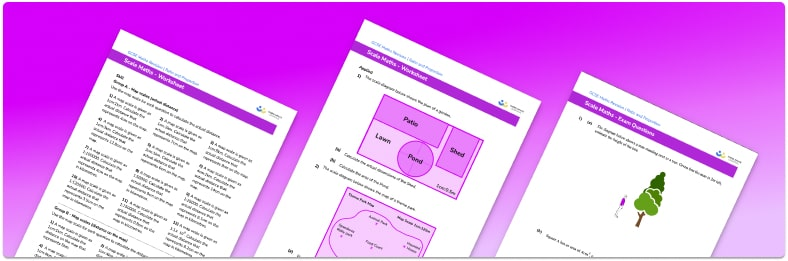# Scale Worksheet• Section 1 of the scale worksheet contains 36 skills-based scale questions, in 3 groups to support differentiation
• Section 2 contains 4 applied scale problems with a mix of worded questions and deeper problem solving questions
• Section 3 contains 4 foundation and higher level GCSE exam style scale questions
• Answers and a mark scheme for all scale questions are provided
• Questions follow variation theory with plenty of opportunities for students to work independently at their own level
• All questions created by fully qualified expert secondary maths teachers
• Suitable for GCSE maths revision for AQA, OCR and Edexcel exam boards

• This field is for validation purposes and should be left unchanged.

You can unsubscribe at any time (each email we send will contain an easy way to unsubscribe). To find out more about how we use your data, see our privacy policy.

### Scale in maths at a glance

Scale in maths involves maps and other scale drawings. If something is drawn to scale, such as a map, it is an accurate representation of a bigger thing, with all distances on the map or drawing in the same proportion as the actual distances in real life.

The map scale is usually given in the form 1:n, where the distances on the map are n times smaller than the actual length in real life. We can calculate missing lengths between two places by measuring the distance on the map and multiplying by n.

For scale drawings and scale diagrams, calculating scale factors can be done by dividing the real distance by the length of the drawing. In the same way, we can calculate the scale factor between two mathematically similar figures by dividing the length of a given side of shape A by the length of the corresponding side of shape B. Once we have the scale factor, it can be used to calculate the lengths of any missing sides of the shapes.

Scale problems require students to be proficient in working with whole numbers, ratio, fractions and decimals, metric units (centimetres, metres, kilometres), reading scales, and conversions. They regularly feature as word problems.

Looking forward, students can then progress to additional ratio and proportion worksheets, for example a ratio worksheet or a simplifying and equivalent ratios worksheet.For more teaching and learning support on Ratio and Proportion our GCSE maths lessons provide step by step support for all GCSE maths concepts.

## Do you have KS4 students who need more focused attention to succeed at GCSE?There will be students in your class who require individual attention to help them succeed in their maths GCSEs. In a class of 30, it’s not always easy to provide.

Help your students feel confident with exam-style questions and the strategies they’ll need to answer them correctly with our dedicated GCSE maths revision programme.

Lessons are selected to provide support where each student needs it most, and specially-trained GCSE maths tutors adapt the pitch and pace of each lesson. This ensures a personalised revision programme that raises grades and boosts confidence.

Find out more